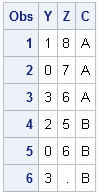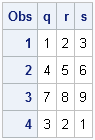In a previous post, I showed how to read data from a SAS data set into SAS/IML matrices or vectors. This article shows the converse: how to use the CREATE, APPEND, and CLOSE statements to create a SAS data set from data stored in a matrix or in vectors.

### Creating a SAS Data Set from SAS/IML Vectors

You can use the CREATE and APPEND statements to write a SAS data set from SAS/IML vectors. The following statements create three different SAS/IML vectors. The data in those vectors are then written to a data set called MyData in the Work library:

```proc iml; /** create SAS data set from vectors **/ y = {1,0,3,2,0,3}; /** 6 x 1 vector **/ z = {8,7,6,5,6}; /** 5 x 1 vector **/ c = {A A A B B B}; /** 1 x 6 character vector **/   create MyData var {y z c}; /** create data set **/ append; /** write data in vectors **/ close MyData; /** close the data set **/```

The CREATE statement opens Work.MyData for writing. The program creates the numerical vectors y and z, and the character vector c. The APPEND statement writes the values of the vectors listed on the VAR clause of the CREATE statement. The numeric vectors create numeric variables; the character vector creates a character variable, which in this case has length 1. The names of the variables in the data set are y, z, and c, which are the same names as the SAS/IML vectors.

The CLOSE statement closes the data set. It is a good programming practice to close data sets when you are finished writing the data.

Notice that it does not matter if a vector is a row vector or a column vector: each element of a vector is written to an observation in the data set. If the vectors do not contain the same number of elements, then the variables that result from the shorter vectors are padded with missing values. For example, the last observation for the z variable contains a missing value, as shown by the following call to PROC PRINT:

`proc print data=MyData; run;`### Creating a SAS Data Set from a SAS/IML Matrix

Use the FROM clause on the CREATE and APPEND statements to create a data set from a matrix. You can specify names for the data set variables by using the COLNAME= option to the FROM clause, as shown in the following example:

```proc iml; /** create SAS data set from a matrix **/ x = {1 2 3, 4 5 6, 7 8 9, 3 2 1 }; /** 4 x 3 matrix **/ create MyData2 from x[colname={"q" "r" "s"}]; append from x; close MyData2;```

For this example, the names of the variables in the data set are q, r, and s. If you do not explicitly specify names for the data set variables, the variables are named COL1, COL2, and so forth.

`proc print data=MyData2; run;`For more details on reading and writing data in the SAS/IML language, see Chapter 3 of Statistical Programming with SAS/IML Software.

ShareDistinguished Researcher in Computational Statistics

Rick Wicklin, PhD, is a distinguished researcher in computational statistics at SAS and is a principal developer of SAS/IML software. His areas of expertise include computational statistics, simulation, statistical graphics, and modern methods in statistical data analysis. Rick is author of the books Statistical Programming with SAS/IML Software and Simulating Data with SAS.

1.Hi Rick,

An example of dealing with coordinate format would be useful.

Thanks,
Leo.

•I don't know what "coordinate format" is, or why it is different than any other matrix.

2.Hi Rick,

Would you be able to give us an example of how you would use the create and append if a do loop
where the data set name is different for each.

For example:

proc iml;
/** create SAS data set from a matrix **/

Do i = 1 to 5;

x = {1 2 3, 4 5 6, 7 8 9, 3 2 1 }; /** 4 x 3 matrix **/
p = x*i;

create MyData_&i from x[colname={"q" "r" "s"}];
append from p;
close MyData_&i;

So the resulting data sets would be:

MyData_1
MyData_2
MyData_3
MyData_4
MyData_5

Thanks,

Scott

3.Worked like a charm. Thanks Rick. Here's what I did in case others need it.

Proc iml;
PCOR = {1.00 0.40 0.40,
0.40 1.00 0.40,
0.40 0.40 1.00};

dsNames = {A B C}; /* specify names of data sets */
do i = 1 to ncol(dsNames);
pvar = diag(vecdiag(pcor))*i;
create(dsNames[i]) from pvar; /* create work.A, then work.B, and so on */
append from pvar;
close (dsNames[i]);
end;

4.Colwel

I run above program but there are some errors pls guide me

571 Proc iml;
572 PCOR = {1.00 0.40 0.40,
573 0.40 1.00 0.40,
574 0.40 0.40 1.00};
575 dsNames={A B C};
575! /* specify names of data sets */
576 do i = 1 to 5;
577 pvar = diag(vecdiag(pcor))*i;
578 create (dsNames[i]) from pvar;
-
22
76
ERROR 22-322: Expecting a name.

ERROR 76-322: Syntax error, statement will be ignored.

579 append from pvar;
580 close (dsNames[i]);
-
22
200
ERROR 22-322: Syntax error, expecting one of the following: a name, ;.

ERROR 200-322: The symbol is not recognized and will be ignored.

581 end;

•You can ask programming questions at the SAS/IML Support Community. Please include your SAS version, since it looks like you might be running an ancient version of SAS. You can obtain your version information by running this SAS statement:
%put SYSVLONG = &SYSVLONG;

•But my problem is related to earlier discussion on this page and I am running following program as mentioned by colwel

But facing some error and not getting out put
my sas version is 9.02

kindly guide me
thanks

•Your version of SAS is more than 10 years old. The feature you are trying to use (reading SAS data sets at run time) is a feature in SAS/IML 12.1.

•I am using SAS 9.3 but still following program is not running

Proc iml;
PCOR = {1.00 0.40 0.40,
0.40 1.00 0.40,
0.40 0.40 1.00};
dsNames = {A B C}; /* specify names of data sets */
do i = 1 to ncol(dsNames);
pvar = diag(vecdiag(pcor))*i;
create(dsNames[i]) from pvar; /* create work.A, then work.B, and so on */
append from pvar;
close (dsNames[i]);
end;

Error:
5078 create(dsNames[i]) from pvar;
-
22
76
ERROR 22-322: Expecting a name.

•You have asked the same question many times and have gotten the answer many times: The feature you are trying to use (reading SAS data sets at run time) is a feature in SAS/IML 12.1, which was released with SAS 9.3m2. Please ask questions like this on the SAS/IML Support Community.

5.Does IML allow for matrices to have different counts by row? That is, something like this:

X = {1 2 3,
2 4,
3 4 5};
•